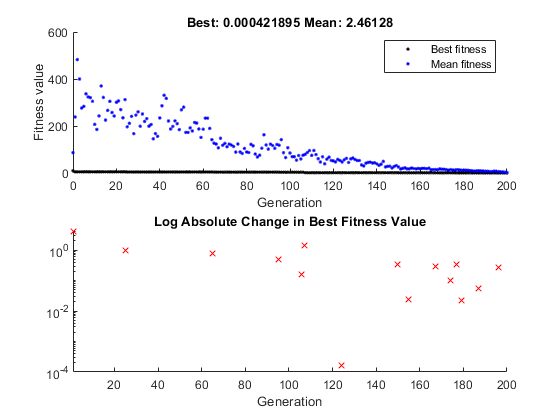Documentation

### This is machine translation

Mouseover text to see original. Click the button below to return to the English version of the page.

## Create Custom Plot Function

If none of the plot functions that come with the software is suitable for the output you want to plot, you can write your own custom plot function, which the genetic algorithm calls at each generation to create the plot. This example shows how to create a plot function that displays the change in the best fitness value from the previous generation to the current generation.

### Creating the Custom Plot Function

To create the plot function for this example, copy and paste the following code into a new file in the MATLAB® Editor.

```function state = gaplotchange(options, state, flag) % GAPLOTCHANGE Plots the logarithmic change in the best score from the % previous generation. % persistent last_best % Best score in the previous generation if(strcmp(flag,'init')) % Set up the plot xlim([1,options.MaxGenerations]); axx = gca; axx.Yscale = 'log'; hold on; xlabel Generation title('Log Absolute Change in Best Fitness Value') end best = min(state.Score); % Best score in the current generation if state.Generation == 0 % Set last_best to best. last_best = best; else change = last_best - best; % Change in best score last_best = best; if change > 0 % Plot only when the fitness improves plot(state.Generation,change,'xr'); end end```

Then save the file as `gaplotchange.m` in a folder on the MATLAB path.

### Using the Plot Function

To use the custom plot function, select Custom in the Plot functions pane and enter `@gaplotchange` in the field to the right. To compare the custom plot with the best fitness value plot, also select Best fitness.Now, if you run the example described in Minimize Rastrigin's Function, the tool displays plots similar to those shown in the following figure.The plot only shows changes that are greater than 0, which are improvements in best fitness. The logarithmic scale enables you to see small changes in the best fitness function that the upper plot does not reveal.

### How the Plot Function Works

The plot function uses information contained in the following structures, which the genetic algorithm passes to the function as input arguments:

• `options` — The current options settings

• `state` — Information about the current generation

• `flag` — Current status of the algorithm

The most important lines of the plot function are the following:

• `persistent last_best`

Creates the persistent variable `last_best`—the best score in the previous generation. Persistent variables are preserved over multiple calls to the plot function.

• `xlim([1,options.MaxGenerations]);`

`axx = gca;`

`axx.Yscale = 'log';`

Sets up the plot before the algorithm starts. `options.MaxGenerations` is the maximum number of generations.

• `best = min(state.Score)`

The field `state.Score` contains the scores of all individuals in the current population. The variable `best` is the minimum score. For a complete description of the fields of the structure state, see Structure of the Plot Functions.

• `change = last_best - best`

The variable change is the best score at the previous generation minus the best score in the current generation.

• `if change > 0`

Plot only if there is a change in the best fitness.

• `plot(state.Generation,change,'xr')`

Plots the change at the current generation, whose number is contained in `state.Generation`.

The code for `gaplotchange` contains many of the same elements as the code for `gaplotbestf`, the function that creates the best fitness plot.

Watch now